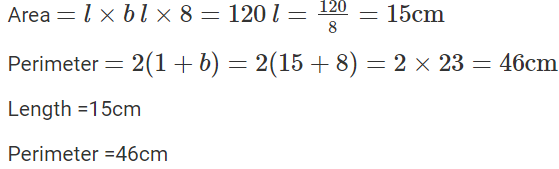# Selina Solutions Concise Maths Class 8 Chapter 20: Area of Trapezium and Polygon

Selina Solutions Concise Maths Class 8 Chapter 20 Area of Trapezium and Polygon provides students with accurate answers to the textbook problems in a systematic manner. A set of highly experienced faculty at BYJU’S curate these solutions with the aim of improving the knowledge about the basic concepts among students. In order to obtain a clear view about the concepts explained here, students can download the ICSE Selina Solutions Class 8 Maths Chapter 20 Area of Trapezium and Polygon PDF, from the link provided below.

From this chapter, it will be easy for the students to understand the method of determining the area, height, perimeter and length of the given figures. Regularly solving the textbook questions using these solutions will enhance the problem solving skills among the students, which is important from the exam perspective.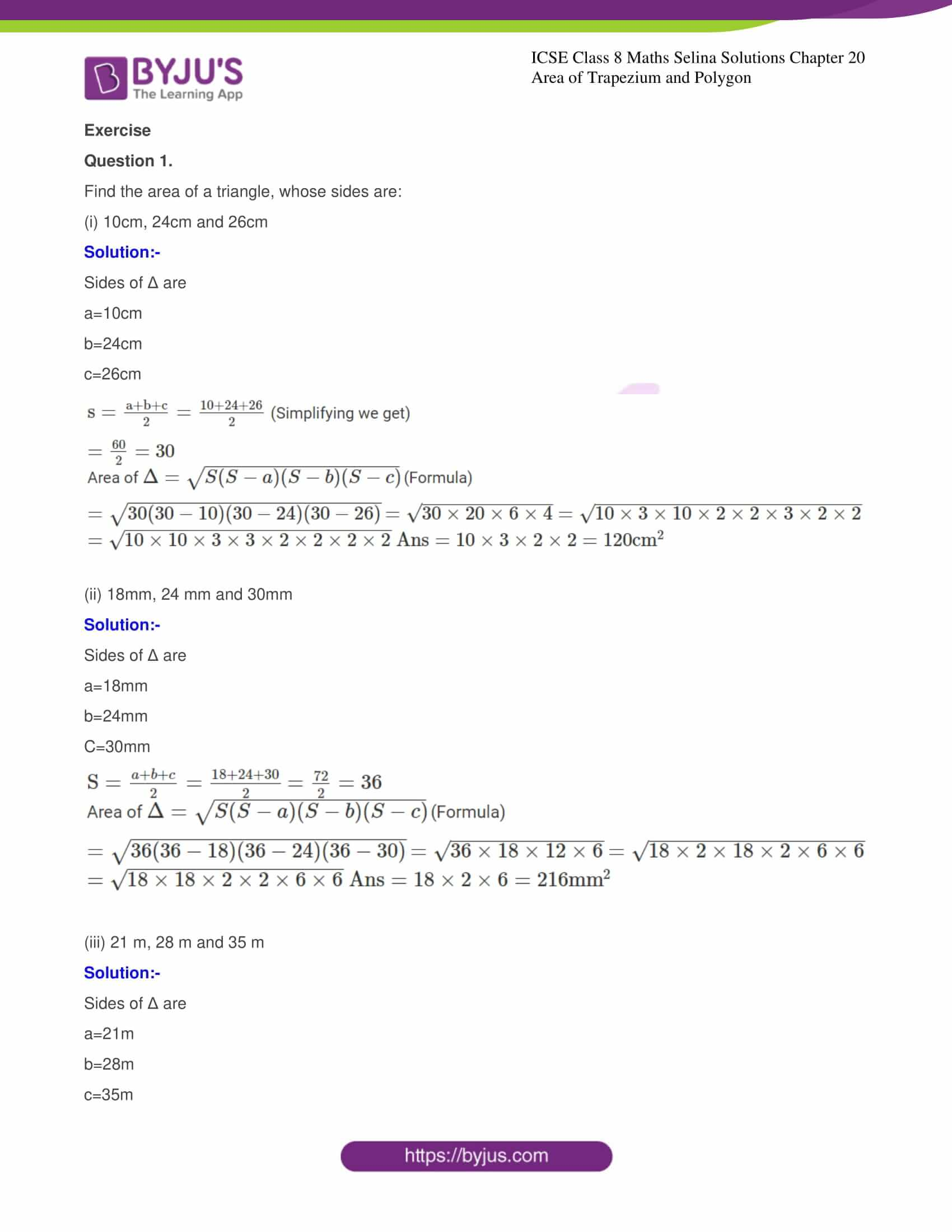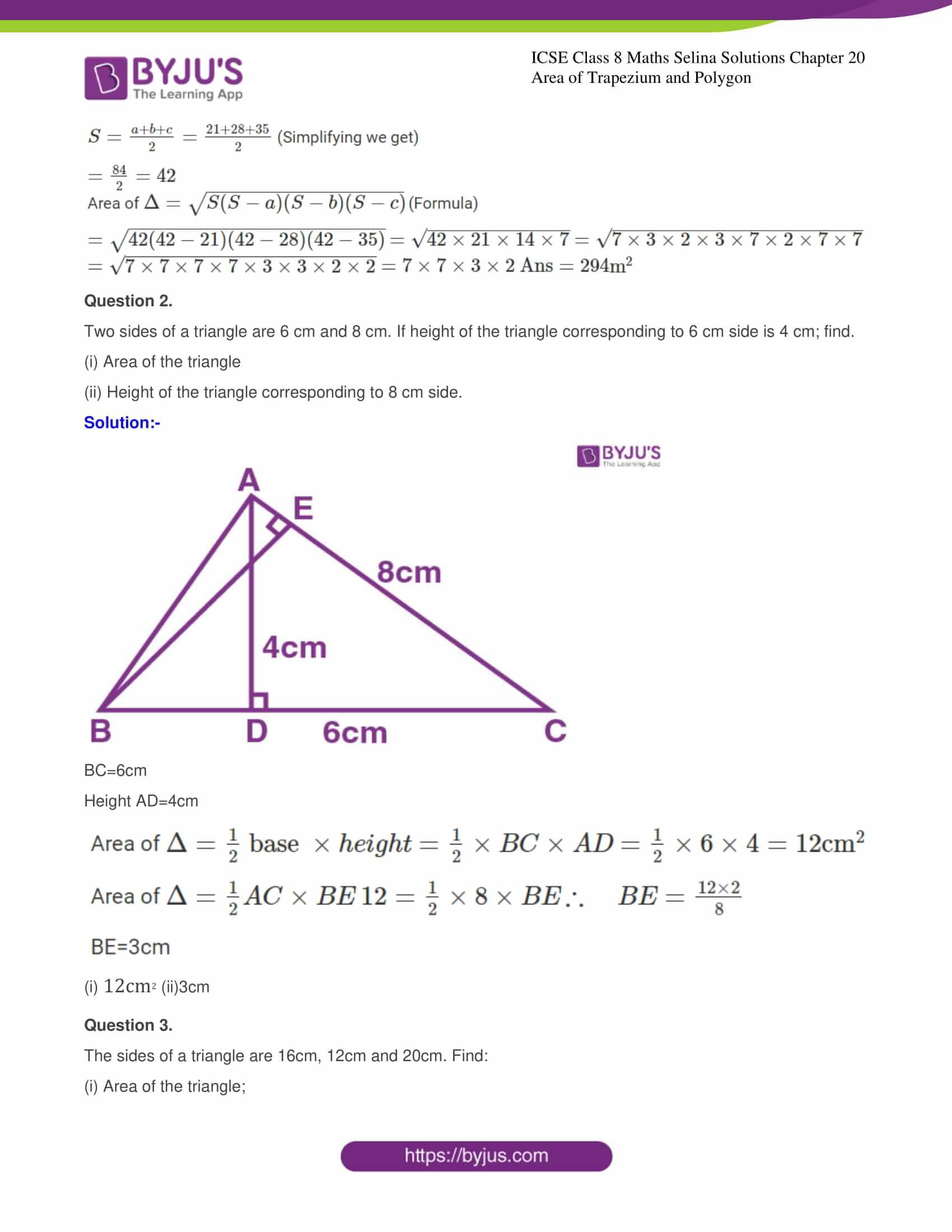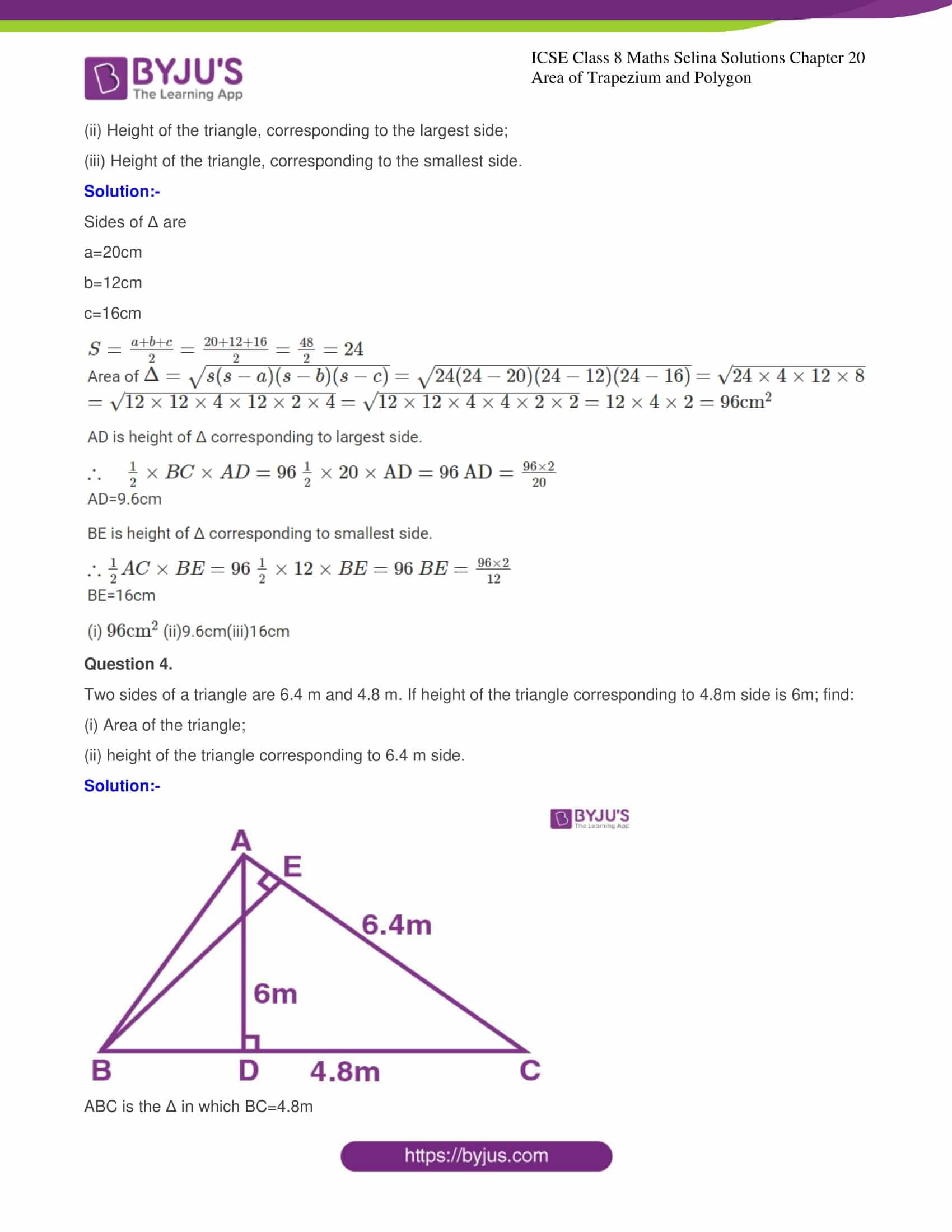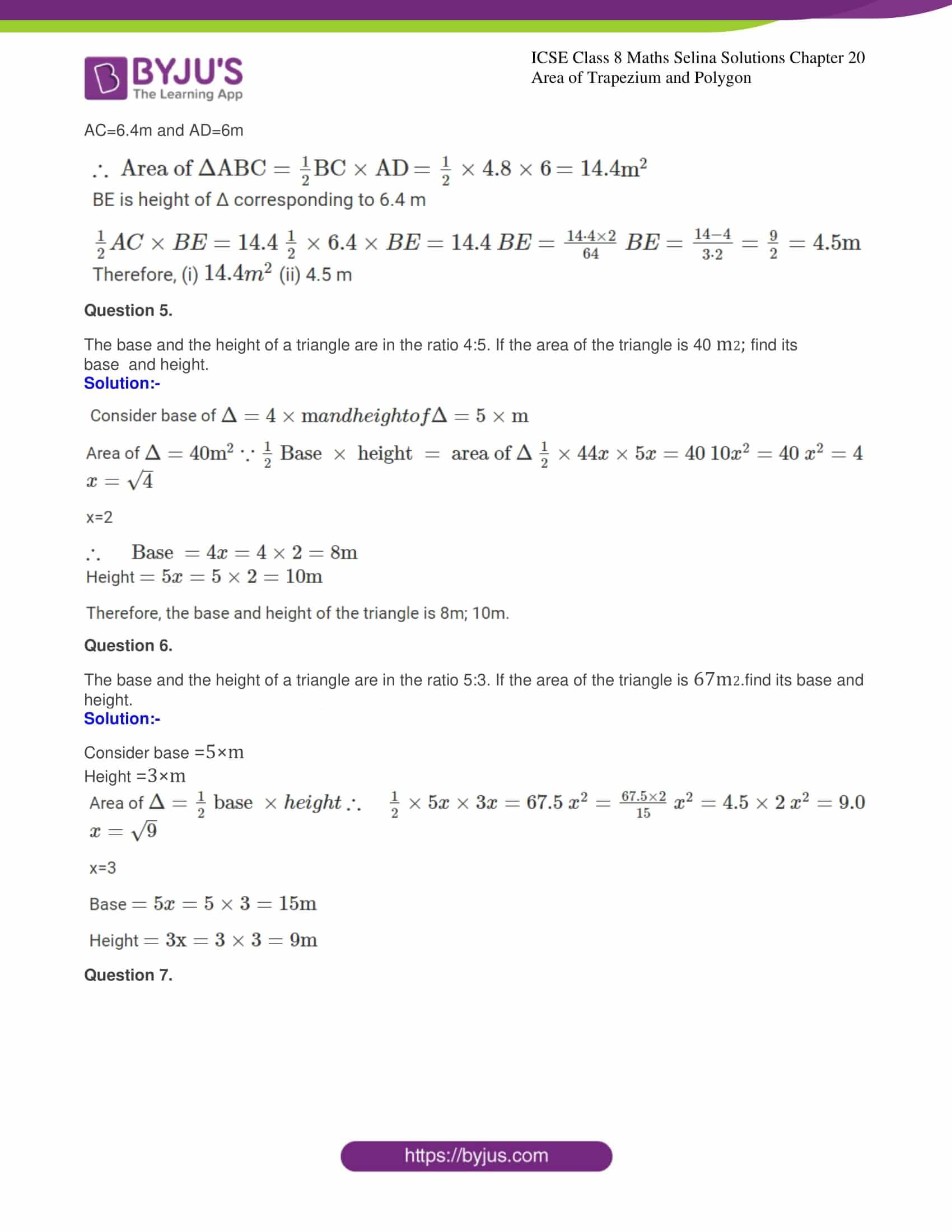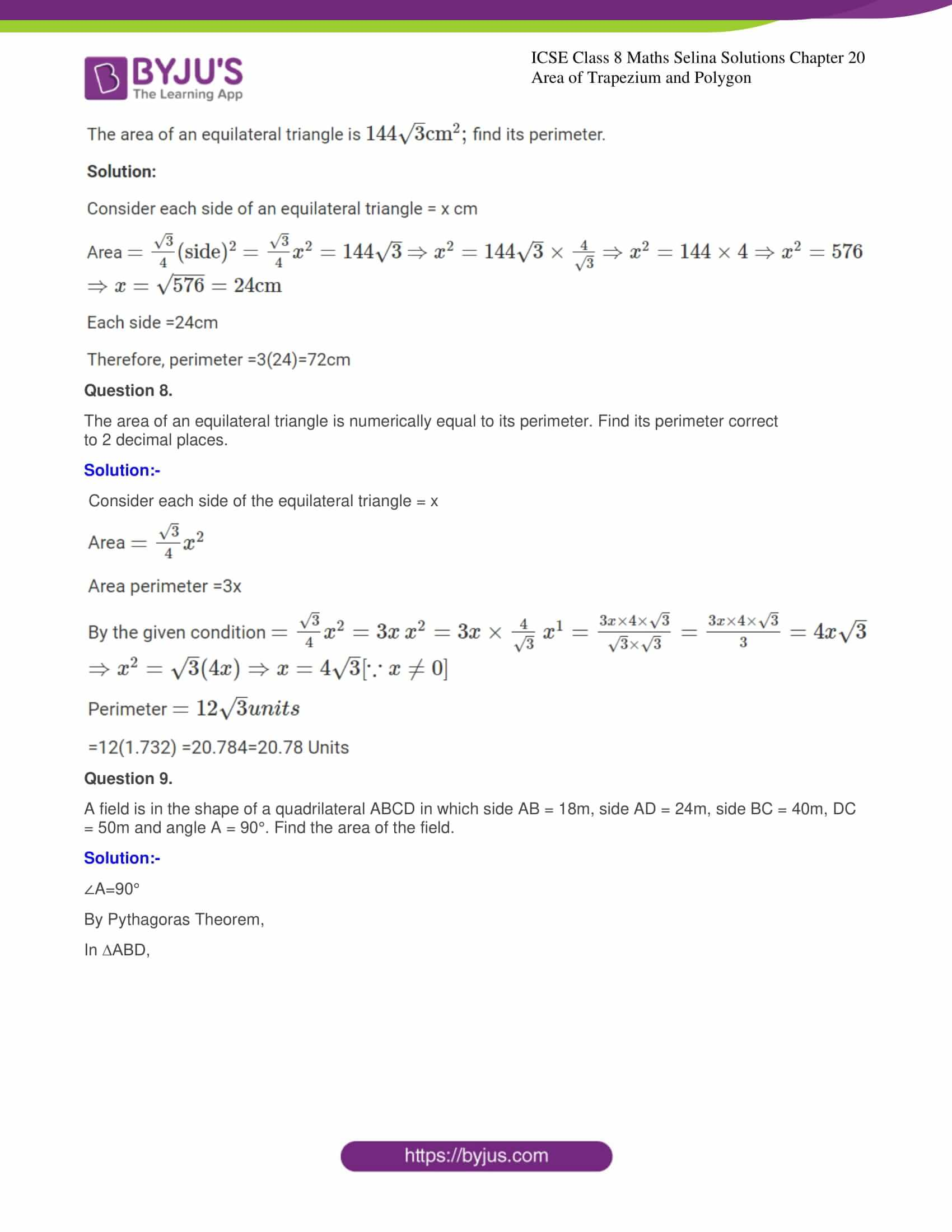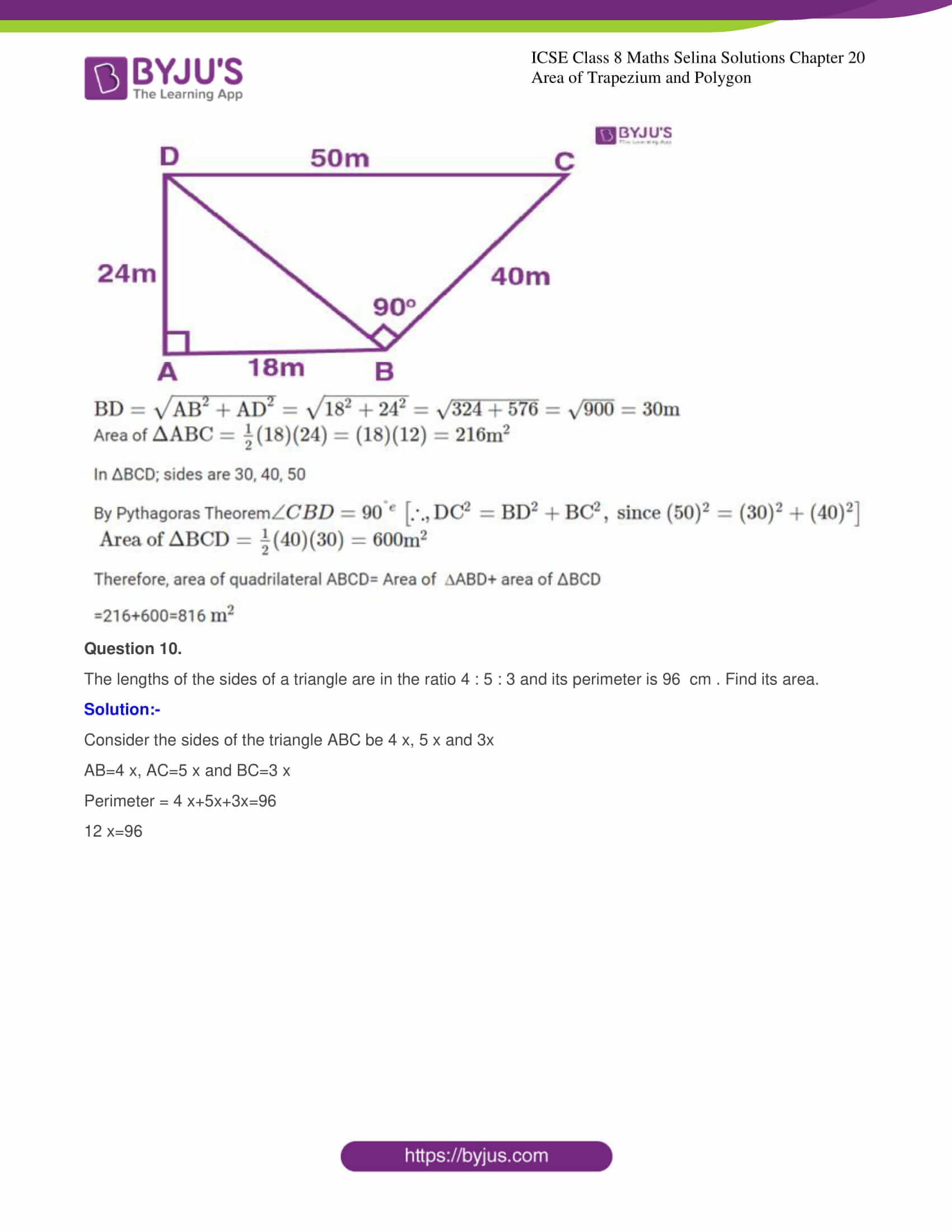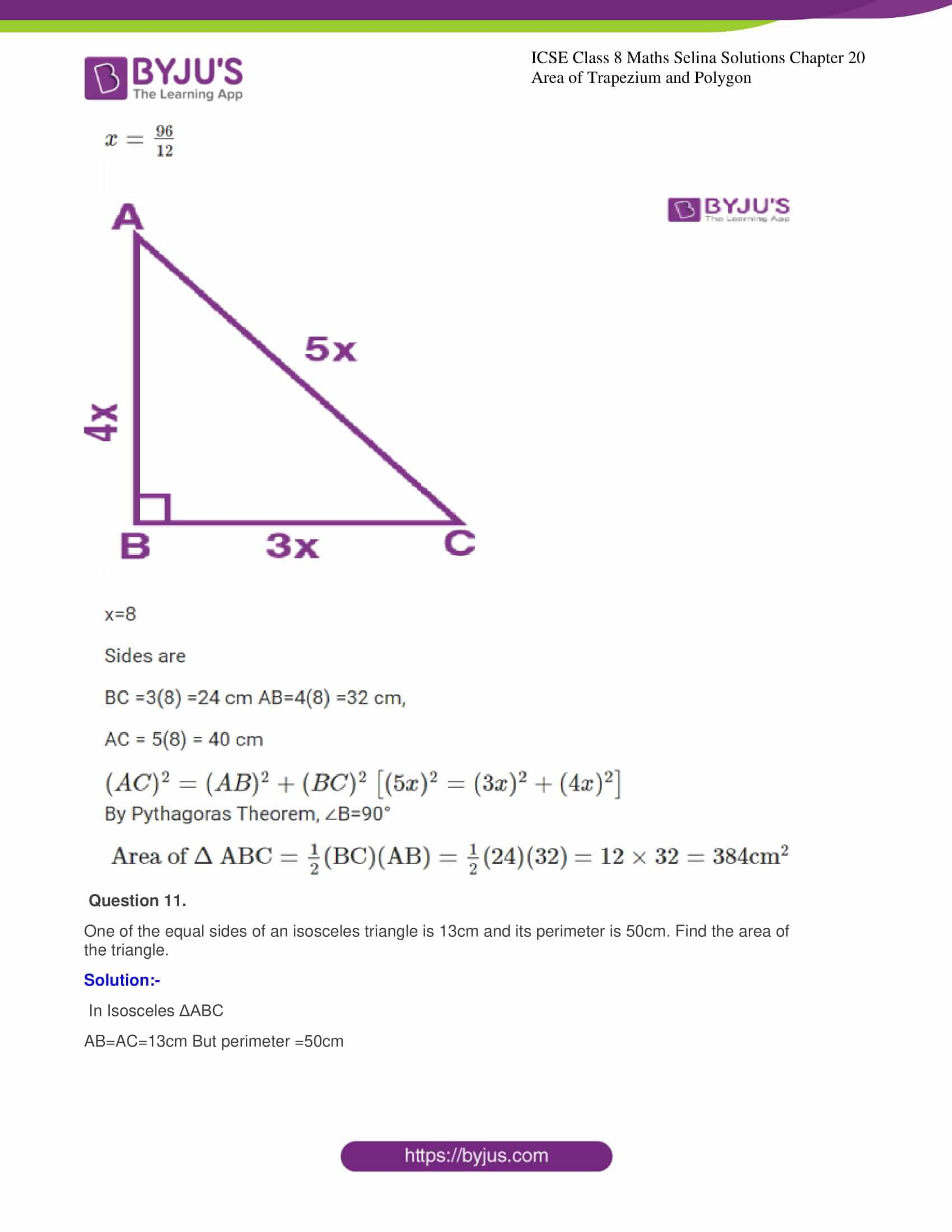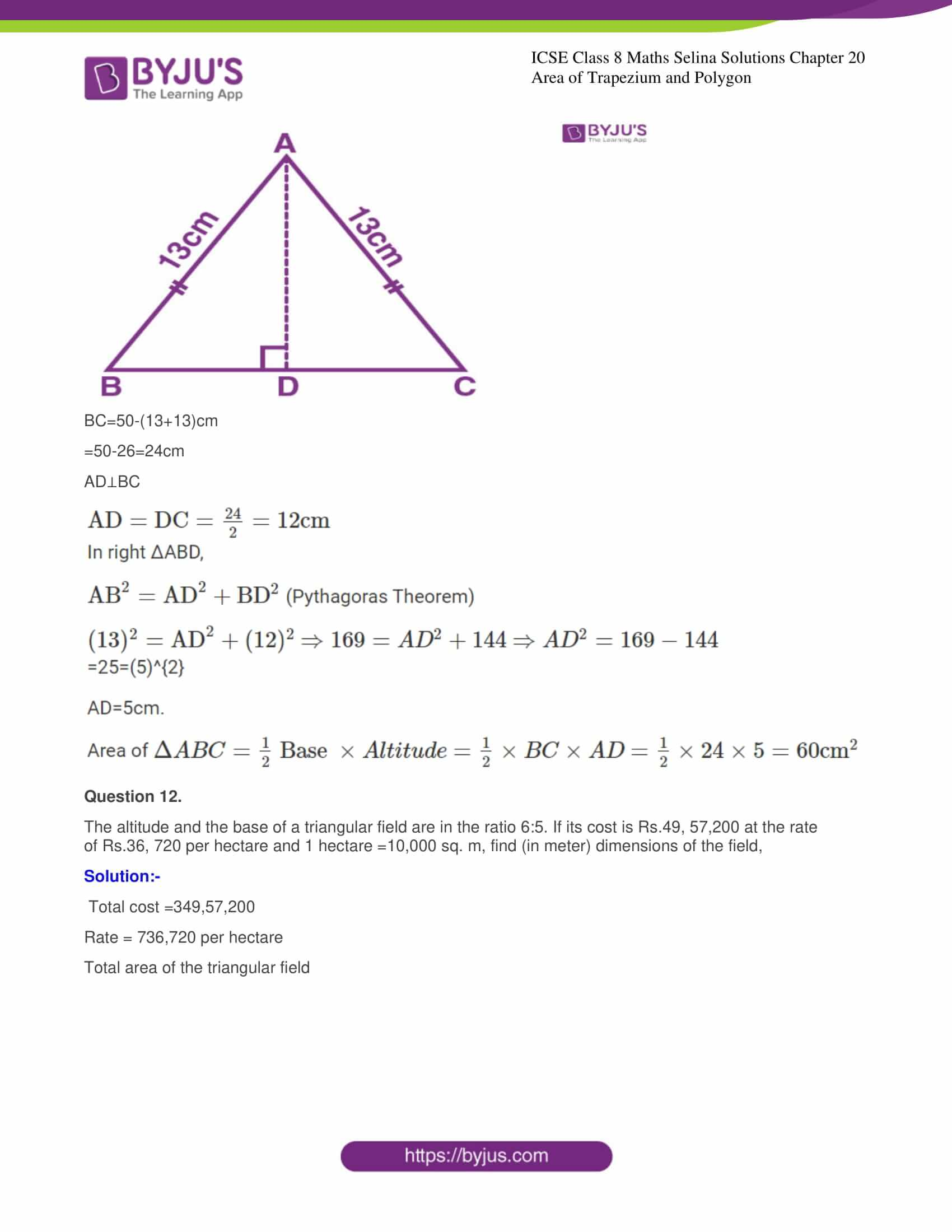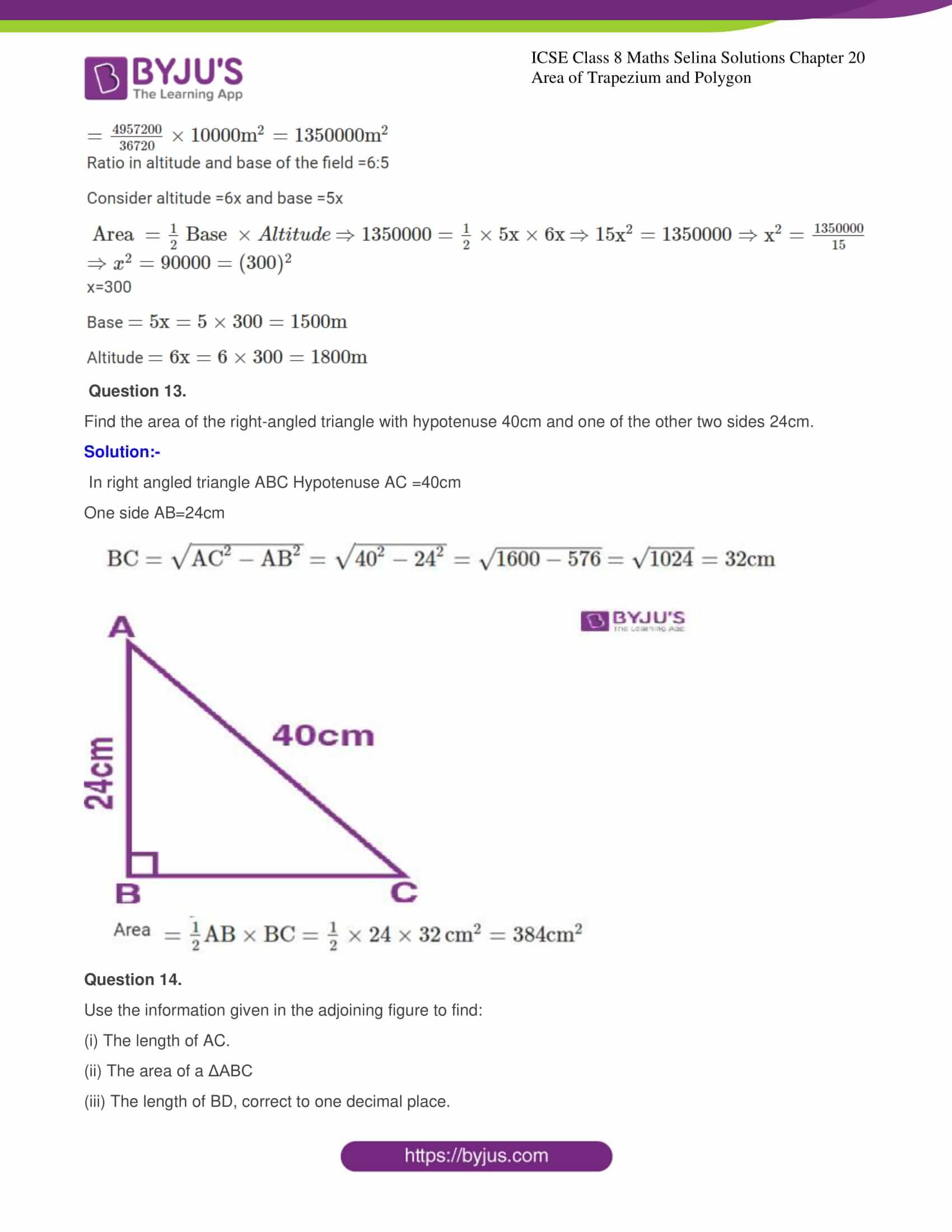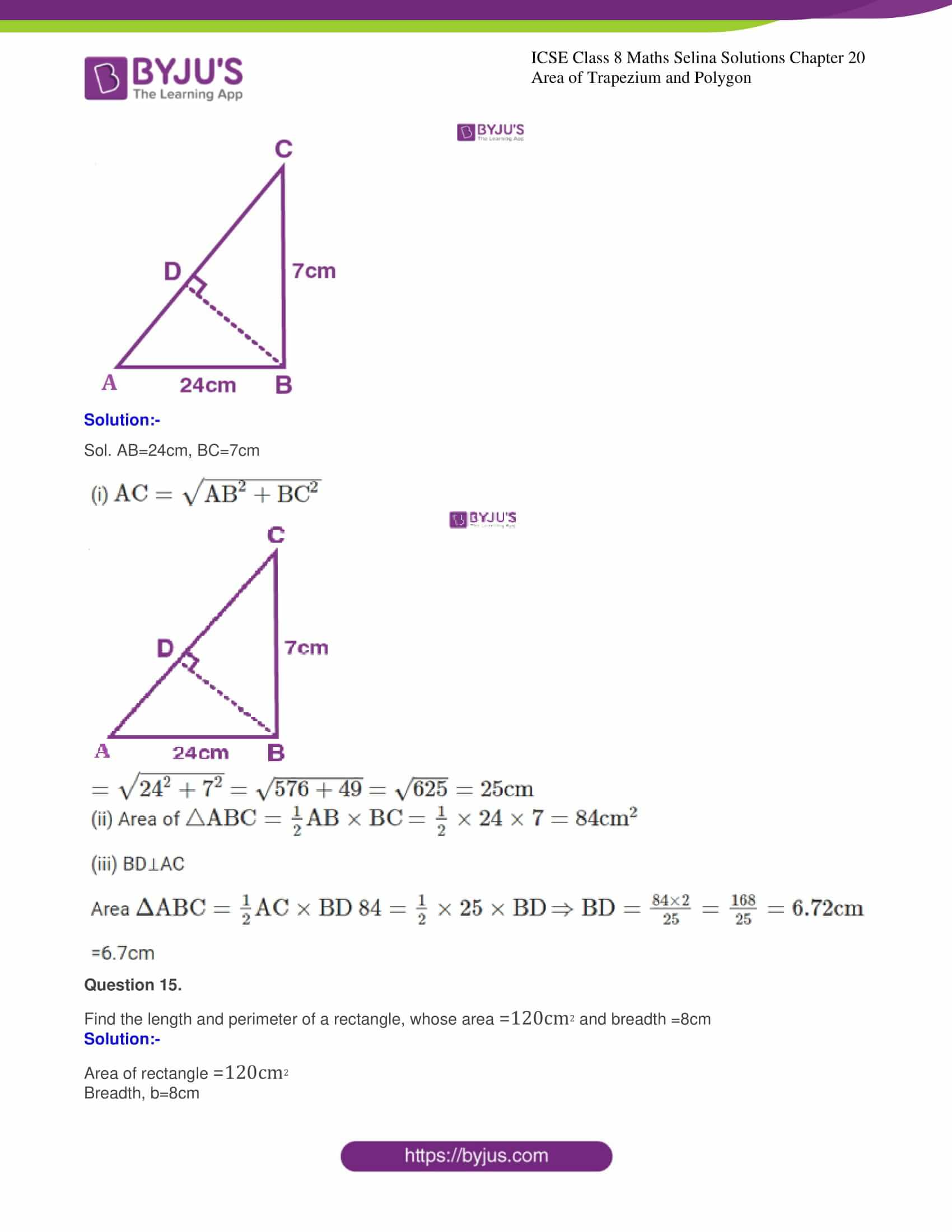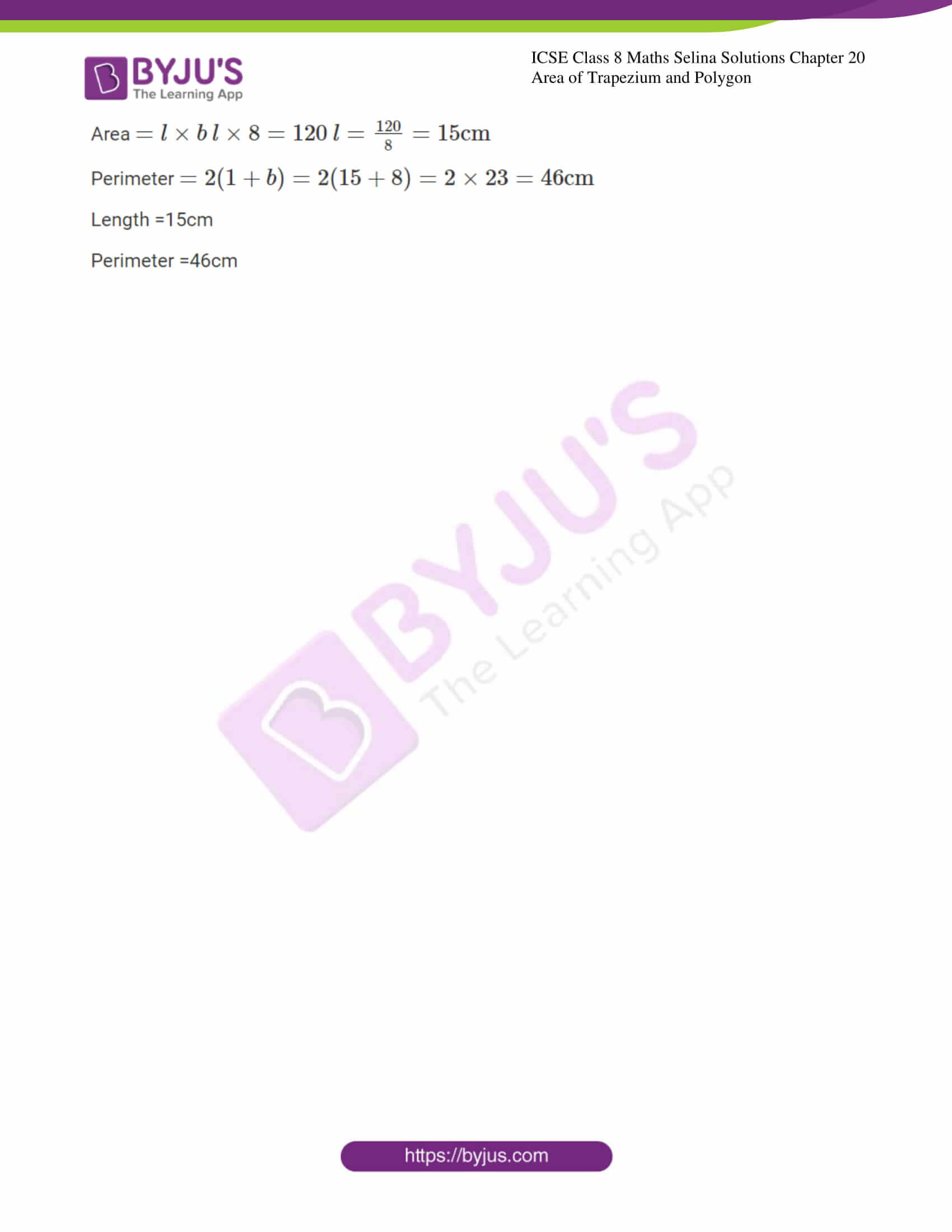## Access Selina Solutions Concise Maths Class 8 Chapter 20: Area of Trapezium and Polygon

Exercise

Question 1.

Find the area of a triangle, whose sides are:

(i) 10cm, 24cm and 26cm

Solution:-

Sides of Δ are

a=10cm

b=24cm

c=26cm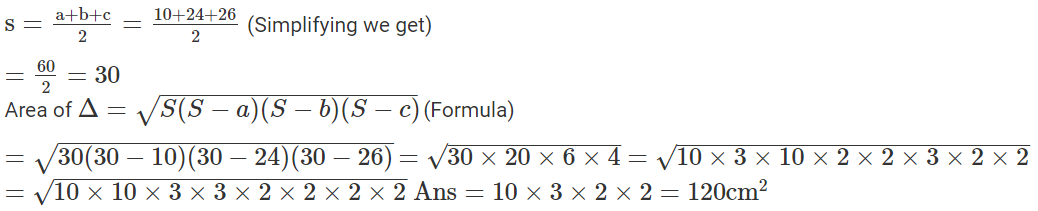(ii) 18mm, 24 mm and 30mm

Solution:-

Sides of Δ are

a=18mm

b=24mm

C=30mm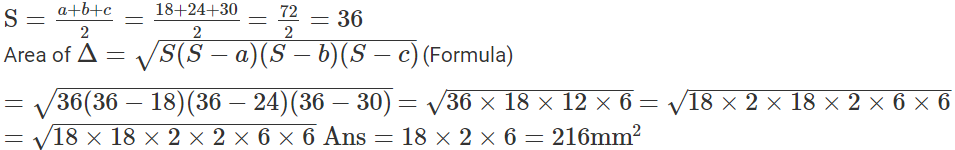(iii) 21 m, 28 m and 35 m

Solution:-

Sides of Δ are

a=21m

b=28m

c=35m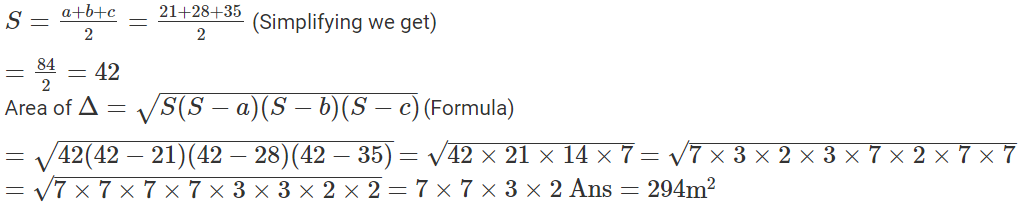Question 2.

Two sides of a triangle are 6 cm and 8 cm. If height of the triangle corresponding to 6 cm side is 4 cm; find.

(i) Area of the triangle

(ii) Height of the triangle corresponding to 8 cm side.

Solution:-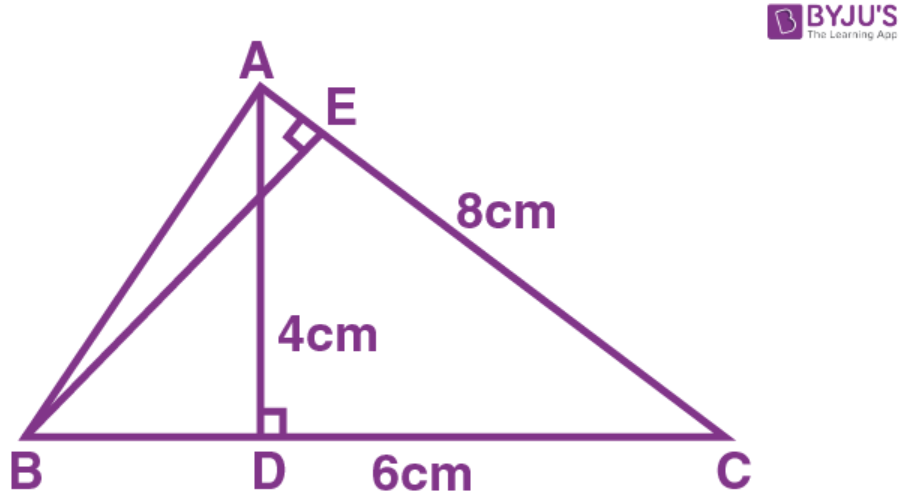BC=6cm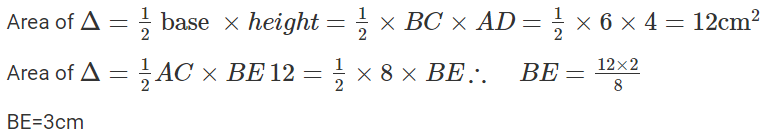(i) 12cm2 (ii)3cm

Question 3.

The sides of a triangle are 16cm, 12cm and 20cm. Find:

(i) Area of the triangle;

(ii) Height of the triangle, corresponding to the largest side;

(iii) Height of the triangle, corresponding to the smallest side.

Solution:-

Sides of Δ are

a=20cm

b=12cm

c=16cm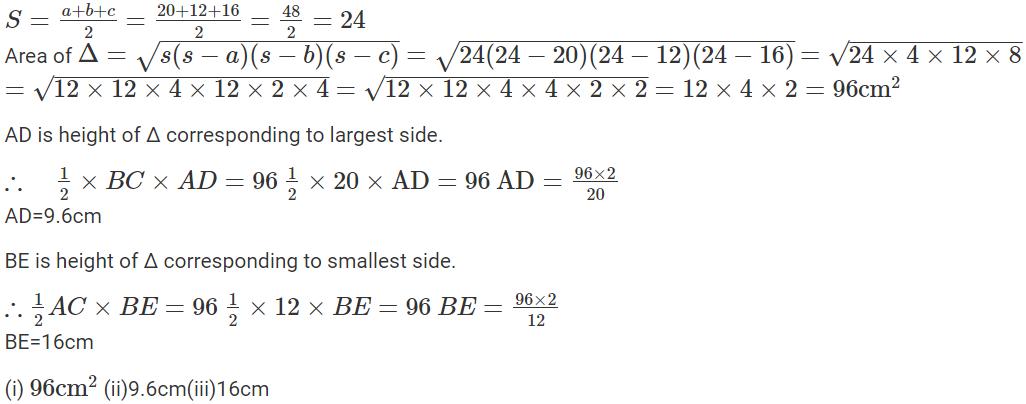Question 4.

Two sides of a triangle are 6.4 m and 4.8 m. If height of the triangle corresponding to 4.8m side is 6m; find:

(i) Area of the triangle;

(ii) height of the triangle corresponding to 6.4 m side.

Solution:-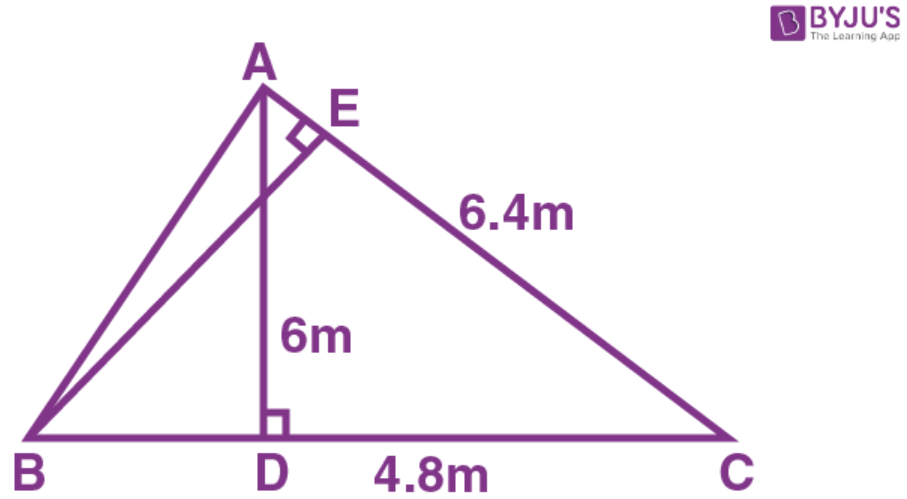ABC is the Δ in which BC=4.8m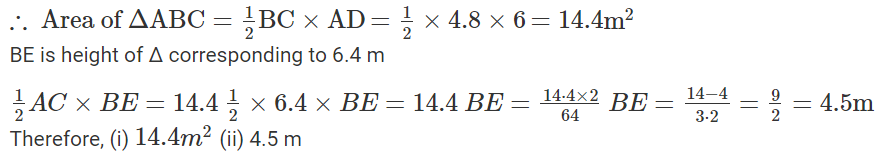Question 5.

The base and the height of a triangle are in the ratio 4:5. If the area of the triangle is 40 m2; find its base  and height.

Solution:-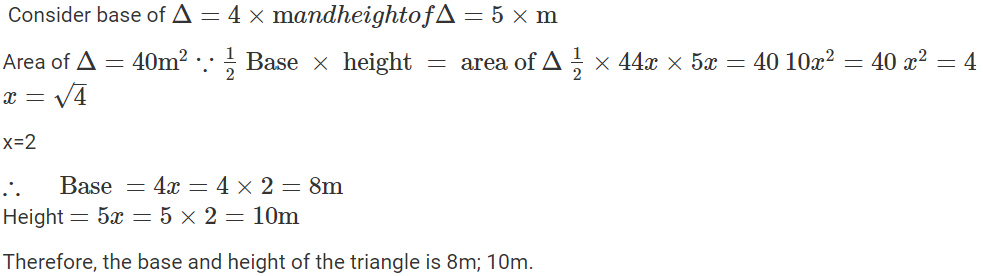Question 6.

The base and the height of a triangle are in the ratio 5:3. If the area of the triangle is 67m2.find its base and height.

Solution:-

Consider base =5×m

Height =3×m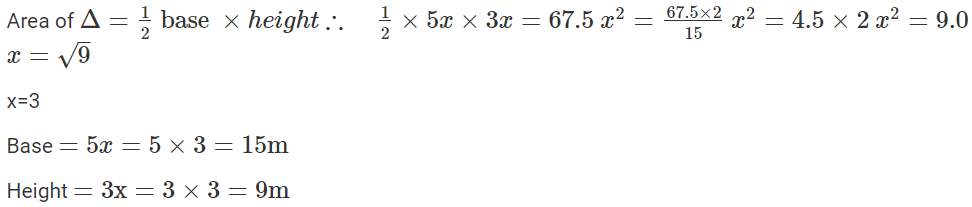Question 7.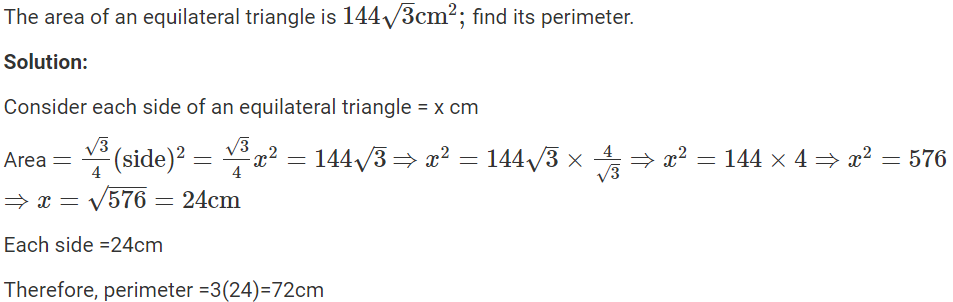Question 8.

The area of an equilateral triangle is numerically equal to its perimeter. Find its perimeter correct to 2 decimal places.

Solution:-

Consider each side of the equilateral triangle = x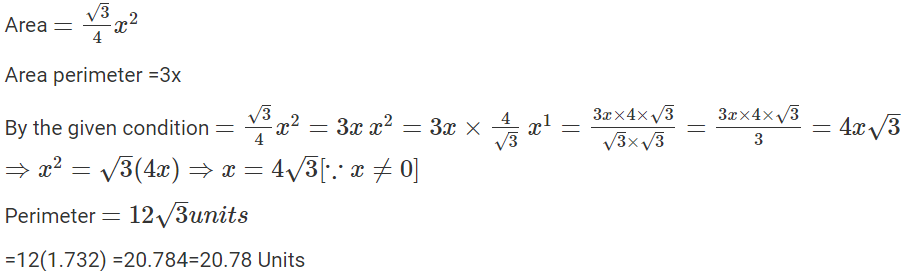Question 9.

A field is in the shape of a quadrilateral ABCD in which side AB = 18m, side AD = 24m, side BC = 40m, DC = 50m and angle A = 90°. Find the area of the field.

Solution:-

∠A=90°

By Pythagoras Theorem,

In ∆ABD,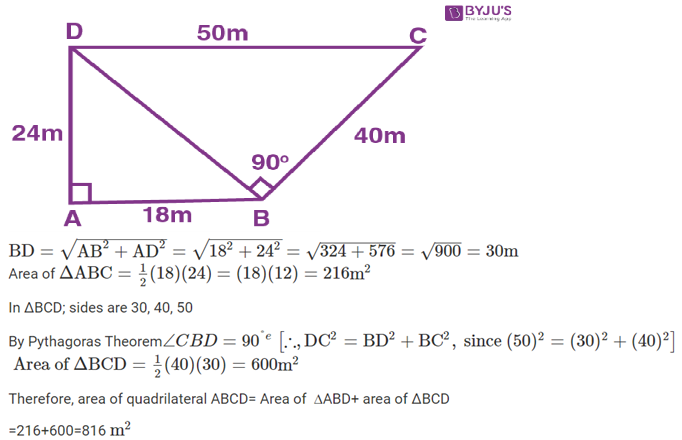Question 10.

The lengths of the sides of a triangle are in the ratio 4 : 5 : 3 and its perimeter is 96  cm . Find its area.

Solution:-

Consider the sides of the triangle ABC be 4 x, 5 x and 3x

AB=4 x, AC=5 x and BC=3 x

Perimeter = 4 x+5x+3x=96

12 x=96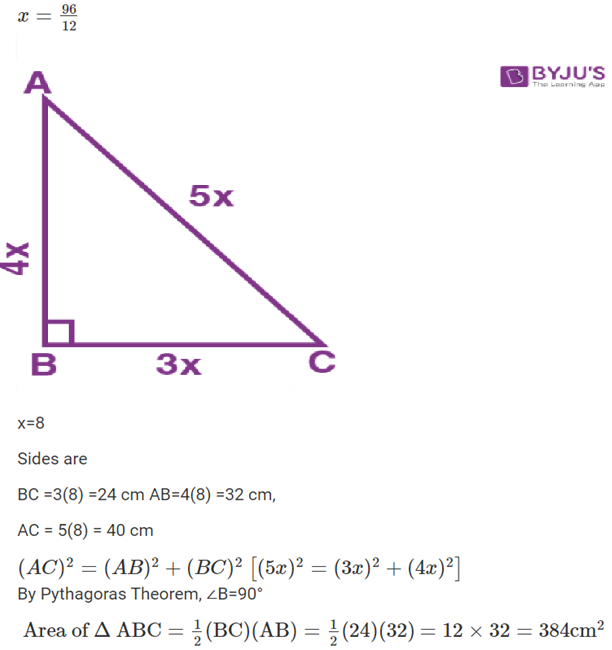Question 11.

One of the equal sides of an isosceles triangle is 13cm and its perimeter is 50cm. Find the area of the triangle.

Solution:-

In Isosceles ΔABC

AB=AC=13cm But perimeter =50cm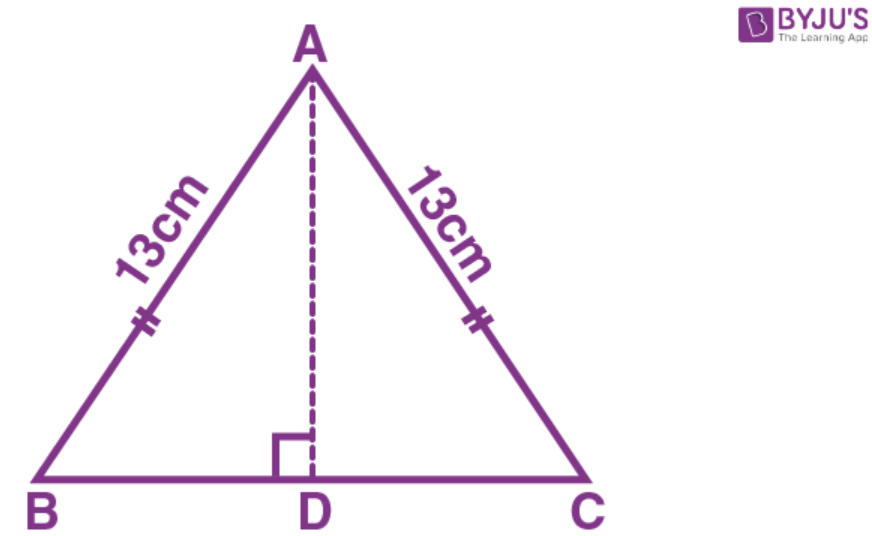BC=50-(13+13)cm

=50-26=24cm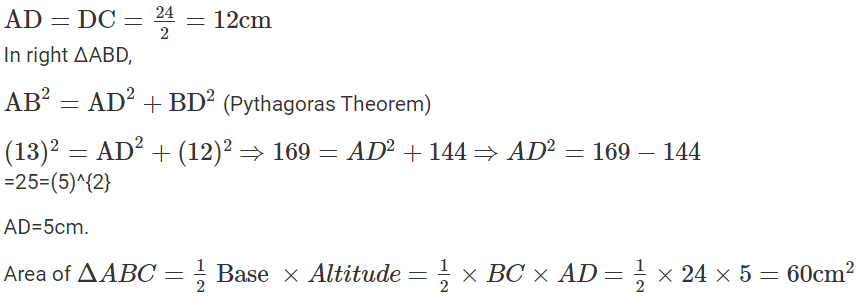Question 12.

The altitude and the base of a triangular field are in the ratio 6:5. If its cost is Rs.49, 57,200 at the rate of Rs.36, 720 per hectare and 1 hectare =10,000 sq. m, find (in meter) dimensions of the field,

Solution:-

Total cost =349,57,200

Rate = 736,720 per hectare

Total area of the triangular field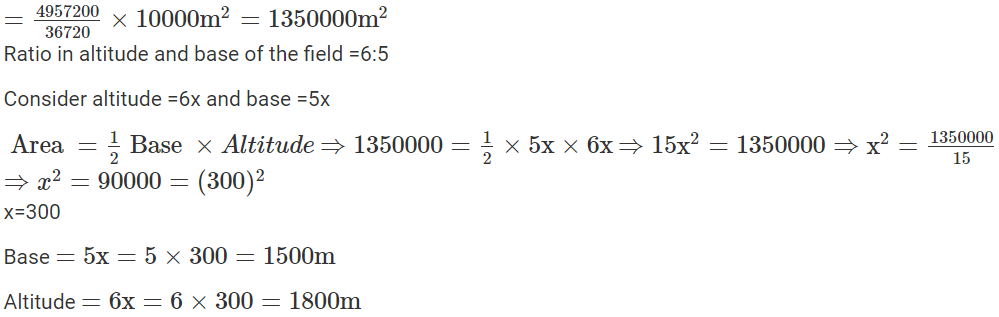Question 13.

Find the area of the right-angled triangle with hypotenuse 40cm and one of the other two sides 24cm.

Solution:-

In right angled triangle ABC Hypotenuse AC =40cm

One side AB=24cm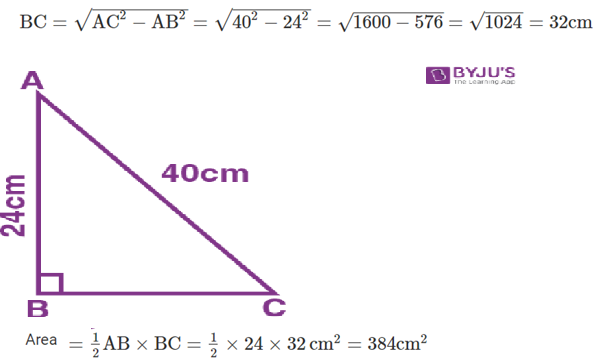Question 14.

Use the information given in the adjoining figure to find:

(i) The length of AC.

(ii) The area of a ΔABC

(iii) The length of BD, correct to one decimal place.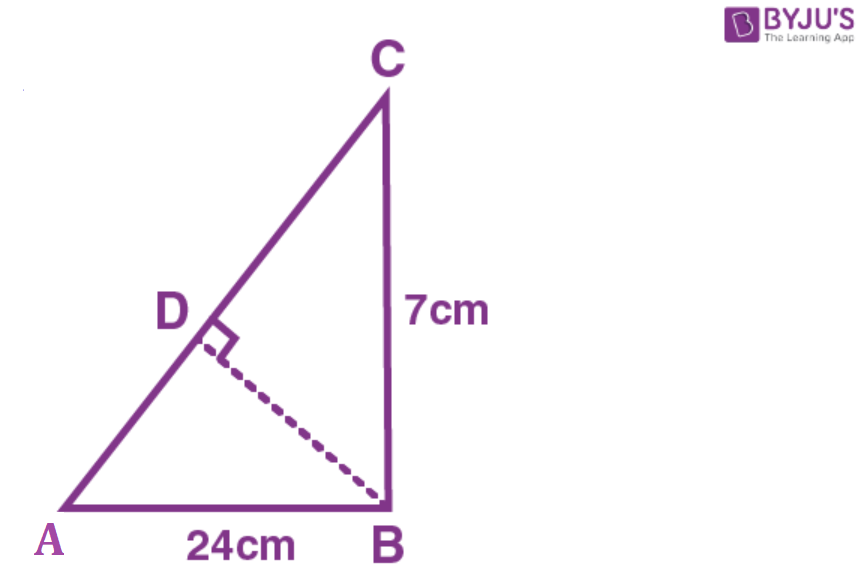Solution:-

Sol. AB=24cm, BC=7cm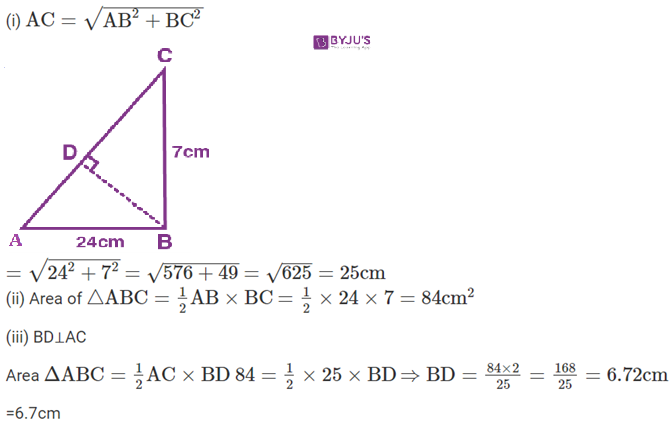Question 15.

Find the length and perimeter of a rectangle, whose area =120cm2 and breadth =8cm

Solution:-

Area of rectangle =120cm2